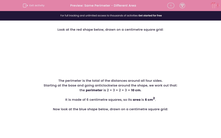# Same Perimeter - Different Area

In this worksheet, students must recognise that shapes with the same perimeters can have different areas.This content is premium and exclusive to EdPlace subscribers.Key stage:  KS 2

Curriculum topic:   Maths and Numerical Reasoning

Curriculum subtopic:   Perimeter and Area

Difficulty level:#### Worksheet Overview

Look at the red shape below, drawn on a centimetre square grid:

The perimeter is the total of the distances around all four sides.

Starting at the base and going anticlockwise around the shape, we work out that:

the perimeter is 2 + 3 + 2 + 3 = 10 cm.

It is made of 6 centimetre squares, so its area is 6 cm2.

Now look at the blue shape below, drawn on a centimetre square grid:

The perimeter is the total of the distances around all its sides.

Starting at the base and going anticlockwise around the shape, we work out that:

the perimeter is 1 + 1 + 1 + 2 + 1 + 1 + 1 + 2 = 10 cm.

This is the same perimeter as the red shape above.

But it is only made of 4 centimetre squares, so its area is only 4 cm2.

Shapes with the same perimeter can have different areas.

### What is EdPlace?

We're your National Curriculum aligned online education content provider helping each child succeed in English, maths and science from year 1 to GCSE. With an EdPlace account you’ll be able to track and measure progress, helping each child achieve their best. We build confidence and attainment by personalising each child’s learning at a level that suits them.

Get started